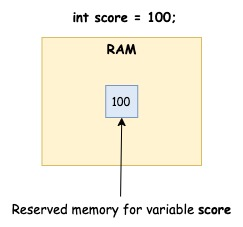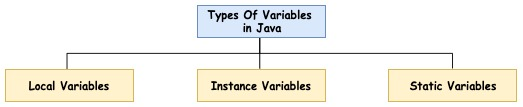# Variables in Java

A variable in java is the name given to a memory location, where a program can store data. Each variable in java is of a specific data type, determines the values it can store, how much memory is required to store this data and the operations that can be performed on it.

In other words, a variable is a name of a memory location. A program can store and manipulate the value stored using variable's identifier.## Rules for Writing Variable Name in Java

Following are the rules for writing names of a variable.

• Variable names in java are case-sensitive. For Example, "Sum" and "sum" will be considered as two different variables.
• ```public class CaseSensitiveVariables {
public static void main(String args[]) {
int sum = 100;
int Sum = 150;
System.out.println("sum : " + sum);
System.out.println("Sum : " + Sum);
}
}
```
Output
```sum : 100
Sum : 150
```
• A variables can be any valid identifier of any length.

• A variable name must start with an alphabet, the dollar sign "\$", or the underscore character "_". A variable names cannot start with a digit. While it's technically legal to begin your variable's name with "_" or "\$", this practice is discouraged. Subsequent characters may be letters, digits, dollar signs, or underscore characters.
• ```int sum;  // Valid variable name.
int _sum; // Valid variable name but not recommended.
int \$sum; // Valid variable name but not recommended.
int 1sum; // InValid variable name.
```
• A java keyword cannot be a variable name.

• White space and special characters and not allowed in variable names.
• ```int su m; // InValid variable name.
int sum'; // InValid variable name.
```

• It is recommended to use full words as variable name instead of cryptic abbreviations. Doing so will make your code easier to read and understand. Field names like counter, sum, and marks are much more intuitive than abbreviated variable names like such i, j, and k.

## Declaration of Variables in Java

Variables in java must be declared before their use in a program. Declaration of a variable associate it with a data type. Here is the syntax for declaring a variable in java :

```data_type variable_name;
```

data_type is one of the data types in java and variable_name is name of variable. variable_name can be any valid identifier.

For Example :
```int sum;
```

We can also initialize a variable at the time of declaration by assigning an initial value.

```int sum = 10;
```

Multiple variables of same data type can be declared by separating the variable names by comma.

```int sum, amount, interest;
int count = 100, total = 40;
```

## Types Of Variables in Java

Java programming language defines three types of variables:### Local Variables

• A local variable is visible only inside their function, only statements inside function can access that local variable.

• Local variables are declared when control enters a function and local variables gets destroyed, when control exits from function.

• Local variables store temporary state inside a method.

• Access modifiers are not used for local variables.

• Local variables are stored n stack.

### Instance Variables

• Instance variables are declared in a class, but outside of any class's methods and constructors.

• Every object of a class will have it's own instance variables.

• Access modifiers are used for instance variables.

• Instance variables get created when a new object is created using new operator and it gets destroyed when object gets destroyed.

• Instance variables are accessibly by all member methods and constructor of a class.

• Instance variables have default values. It is zero for numerical variables, false of boolean variables and null for object references.

### Class/Static Variables

• A class variable is any field declared with the static modifier.

• Only one copy of this variable is created for a class, irrespective of the number of objects created. If we created 10 objects of class C, then all 10 onjects will share same copy of static variable. There will be only one copy of static variable not 10 copies(one for each object).

• Static variables are stored in the static memory. Static variables are created only once at the time class loading.

• Static variables are also known as class variables, as they are associated with a class unlike instance variables which are specific to objects. Static variables can be accessed by calling with the class name ClassName.StaticVariableName. We don't have to create any object to access static variables.